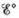# The overall reaction and equilibrium constant value for a hydrogen-oxygen fuel cell at 298 K is 2 H 2 ( g ) + O 2 ( g ) → 2 H 2 O ( l ) K = 1.28 × 10 83 a. Calculate and ∆ G ° at 298 K for the fuel cell reaction. b. Predict the signs of ∆ H ° and ∆ S ° for the fuel cell reaction. c. As temperature increases, does the maximum amount of work obtained from the fuel cell reaction increase, decrease, or remain the same? Explain.### Chemistry: An Atoms First Approach

2nd Edition
Steven S. Zumdahl + 1 other
Publisher: Cengage Learning
ISBN: 9781305079243

#### Solutions

Chapter
Section### Chemistry: An Atoms First Approach

2nd Edition
Steven S. Zumdahl + 1 other
Publisher: Cengage Learning
ISBN: 9781305079243
Chapter 17, Problem 124AE
Textbook Problem
399 views

## The overall reaction and equilibrium constant value for a hydrogen-oxygen fuel cell at 298 K is 2 H 2 ( g )   +   O 2 ( g )   →   2 H 2 O ( l ) K   =   1.28   ×   10 83 a. Calculateand ∆G° at 298 K for the fuel cell reaction.b. Predict the signs of ∆H° and ∆S° for the fuel cell reaction.c. As temperature increases, does the maximum amount of work obtained from the fuel cell reaction increase, decrease, or remain the same? Explain.

(a)

Interpretation Introduction

Interpretation:

The reaction taking place in Hydrogen-Oxygen fuel cell is given. Various questions based on the given reaction have to be answered.

Concept introduction:

A fuel cell is an electrochemical cell that produces electrical energy from the chemical energy. They work as long as the fuel is fed to them and they are eco-friendly.

To determine: The value of E°cell and ΔG° at 298K for the fuel cell reaction.

The value of ΔG° is -474.2kJ_ .

### Explanation of Solution

Given,

The given reaction is,

2H2+O22H2O

The value of K is 1.28×1083 .

The reaction taking place at cathode is,

O2+4H++4e2H2OE°red=1.23V

The reaction taking place at anode is,

2H24H++4eE°ox=0.00V

The relationship between Gibbs free energy change and equilibrium constant is given by the formula,

ΔG°=RTlnK

Where,

• ΔG° is the Gibbs free energy change.
• R is the universal gas constant (8.314×103kJmole1K1) .
• T is the temperature in Kelvin.
• K is the equilibrium constant.

Substitute the values of R , T and K in the above equation as,

ΔG°=RTlnK=(8.314×103kJmole1K1)(298K)×2.303log(1.28×1083)=-474.2kJ_

The value of ΔG° is -474

(b)

Interpretation Introduction

Interpretation:

The reaction taking place in Hydrogen-Oxygen fuel cell is given. Various questions based on the given reaction have to be answered.

Concept introduction:

A fuel cell is an electrochemical cell that produces electrical energy from the chemical energy. They work as long as the fuel is fed to them and they are eco-friendly.

To determine: The sign of ΔH° and ΔS° for the fuel cell reaction and sign of ΔS° is negative.

The sign of ΔH° is negative for the fuel cell reaction.

(c)

Interpretation Introduction

Interpretation:

The reaction taking place in Hydrogen-Oxygen fuel cell is given. Various questions based on the given reaction have to be answered.

Concept introduction:

A fuel cell is an electrochemical cell that produces electrical energy from the chemical energy. They work as long as the fuel is fed to them and they are eco-friendly.

To determine: The affect of increased temperature over the maximum amount of work obtained from the fuel cell reaction.

The maximum amount of work obtained from the fuel cell reaction decreases as the temperature is increased.

### Still sussing out bartleby?

Check out a sample textbook solution.

See a sample solution

#### The Solution to Your Study Problems

Bartleby provides explanations to thousands of textbook problems written by our experts, many with advanced degrees!

Get Started

Find more solutions based on key concepts
What is an atoll and how does it form?

Fundamentals of Physical Geography

To prevent wasting of dietary protein, which of the following conditions must be met? a. Dietary protein must b...

Nutrition: Concepts and Controversies - Standalone book (MindTap Course List)

Name two strategies for increasing a school-age childs physical activity.

Nutrition Through the Life Cycle (MindTap Course List)

Review. The average density of the planet Uranus is 1.27 103 kg/m3. The ratio of the mass of Neptune to that o...

Physics for Scientists and Engineers, Technology Update (No access codes included)

How would this unusual reproductive behavior benefit these small fish?

Oceanography: An Invitation To Marine Science, Loose-leaf Versin